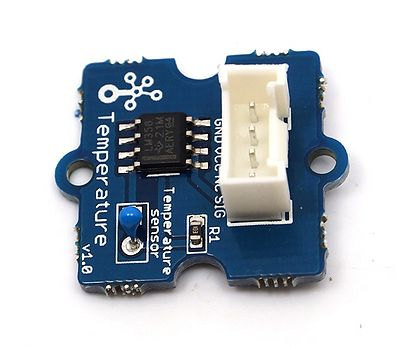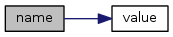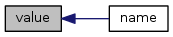upm  1.7.1 Sensor/Actuator repository for libmraa (v2.0.0)
Temperature Class Reference

API for NTC Temperature Sensors. More...

## Detailed Description

Basic UPM module for analog temperature sensors. These sensors use a NTC thermistor to measure ambient temperature. This driver was developed using the Grove Temperature Sensor. The conversion formula has been updated to work with versions 1.1 and 1.2 of the sensor. For the older v1.0 sensor you will have to specify R0 and B values when initializing the device. The range of this sensor is -40 to 125 C and accuracy is +/- 1.5 C.// Create the temperature sensor object using AIO pin 0
std::cout << temp.name() << std::endl;
// Read the temperature ten times, printing both the Celsius and
// equivalent Fahrenheit temperature, waiting one second between readings
for (int i = 0; i < 10; i++) {
int celsius = temp.value();
int fahrenheit = (int) (celsius * 9.0 / 5.0 + 32.0);
printf("%d degrees Celsius, or %d degrees Fahrenheit\n", celsius, fahrenheit);
upm_delay(1);
}
// Delete the temperature sensor object

## Public Member Functions

Temperature (unsigned int pin, float scale=1.0, int r0=100000, int b=4275)

~Temperature ()

float raw_value ()

std::string name ()

int value ()

## Constructor & Destructor Documentation

 Temperature ( unsigned int pin, float scale = `1.0`, int r0 = `100000`, int b = `4275` )

Analog temperature sensor constructor

Parameters
 pin Analog pin to use scale Scaling factor for raw analog value from the ADC, useful for mixed 3.3V/5V boards, default 1.0 r0 zero power resistance, this is 100K (default) for v1.1-v1.2 and 10K for v1.0 of the sensor b thermistor nominal B constant, this is 4275 (default) for v1.1-v1.2 and 3975 for v1.0 of the sensor
 ~Temperature ( )

Analog temperature sensor destructor

## Member Function Documentation

 float raw_value ( )

Gets the raw value from the AIO pin

Returns
Raw value from the ADC
 std::string name ( )
inline

Provided for compatibility with old grove base class

Returns
Sensor name

Here is the call graph for this function:int value ( )

Gets the temperature in Celsius from the sensor

Returns
Normalized temperature in Celsius

Here is the caller graph for this function:The documentation for this class was generated from the following files: